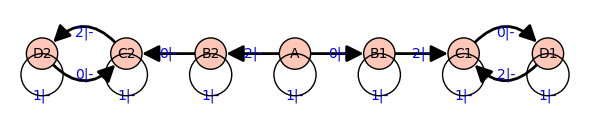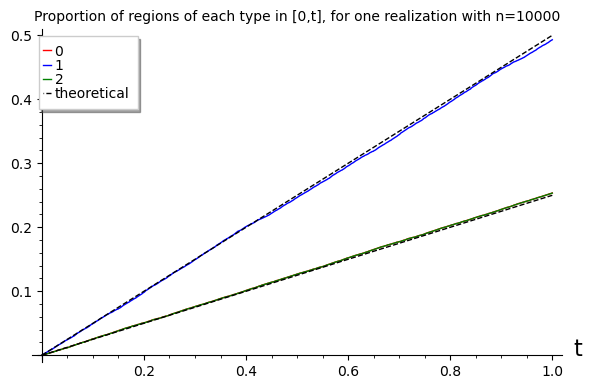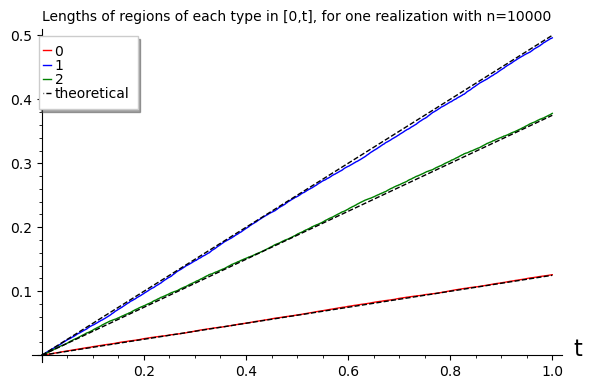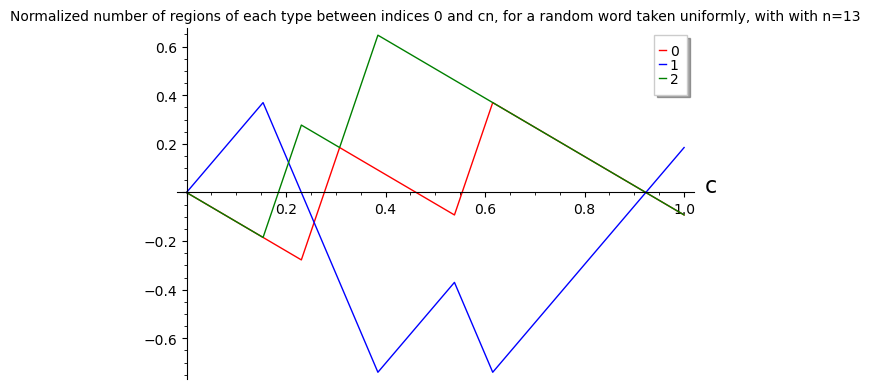# Simulation and illustration of several results of "Points and lines configurations for perpendicular bisectors of convex cyclic polygons''¶

In this notebook we illustrate several of our results, by means of simulation and explicit computation. We follow the subsections, references and order of the revised version of the paper.

In :
# We make the simulations repeatable by setting the seed
set_random_seed(0)


## 1.1 Deterministic results¶

We begin with Theorem 1, which states that a word is realizable iff its signature is interlacing.

In :
def sim_data(n):
# simulates a configuration for n iid points uniform on the circle
# the data is returned as a sorted list of elements of type (pos,index)
# where 'pos' is the position in [0,1] in increasing order, and 'index' is either:
# - 0 for a point
# - 1 for the diametrically opposite point
# - 2 for a perpendicular bisector
# - 3 for the diametrically opposite part of the bisector
P = [random() for _ in range(n)] #n iid points
PP = [(P[i] + 1/2) % 1 for i in range(n)] #diametrically opposite points
P.sort()
L = [ (P[i] + P[i+1]) / 2 for i in range(n-1)] + [ (P[n-1]-1+P)/2 %1 ] #bisectors
LL = [ (L[i] + 1/2) % 1 for i in range(n)] #diametrically opposite side of bisectors
# the next list, U will contain, the data described before
U = [(x,0) for x in P] + [(x,1) for x in PP] + [(x,2) for x in L] + [(x,3) for x in LL]
U.sort() #default sorting is w.r.t. the first entries
return U

In :
def sim_word(n):
# simulates data and deduces the occupancy word
U = sim_data(n)
V = [] # will be the occupancy word
current_number = 0
for (_,x) in U:
if x==2 or x==3:
V.append(current_number)
current_number=0
if x==0:
current_number+=1
V += current_number
return V

# an occupancy word for n=10 random points:
sim_word(10)

Out:
[0, 1, 0, 1, 1, 0, 1, 0, 1, 0, 1, 1, 0, 1, 0, 0, 1, 0, 1, 0]
In :
def word_to_sig(V):
# takes a word and returns its signature
n = len(V)/2
return [V[i] + V[i+n] for i in range(n)]
def sim_sig(n):
# simulates the signature of the occupancy word
V = sim_word(n)
return word_to_sig(V)

# a signature of a word for n=10 random points:
sim_sig(10)

Out:
[1, 0, 2, 1, 0, 1, 1, 1, 2, 1]
In :
# We now test if signatures are always interlacing.
def is_interlacing(S):
# Takes a signature S (list of 0, 1 and 2) and says if it is interlacing
n0 = S.count(0)
n2 = S.count(2)
# If there are no 0, no 2, or not the same number of 0 and 2, fail:
if n0==0 or n0!=n2:
return False
# Otherwise, we run through S and each time we see a 0/2, we check if the previous was different.
# Initially, 'previous' is the one *different* from the first 0/2 in S.
i0 = S.index(0) #first index for 0
i2 = S.index(2) #first index for 2
if i0<i2:
previous=2
else:
previous=0
for x in S:
if x==0 or x==2:
if x==previous:
return False
previous=x
return True

In :
# We simulate n_tests signatures for n, and check if they are all interlacing.
n_tests=1000000
n=20

n_fail=0
for _ in range(n_tests):
if not(is_interlacing(sim_sig(n))):
print('fail')
n_fail += 1

print('Number of non-interlacing signatures found: ' + str(n_fail) )

Number of non-interlacing signatures found: 0


We can now illustrate Proposition 2, which states uniqueness of the occupancy word for $n=3$ up to symmetries.

In :
# occupancy word for a random word with n=3
sim_word(3)

Out:
[1, 0, 0, 1, 0, 1]
In :
# we test that this is indeed the only word up to symmetries (i.e. the only bracelet),
# for n_tests simulations
n_tests = 1000000
n_mistakes = 0
for _ in range(n_tests):
V = sim_word(3)
# we make the word 'canonical' by computing the rotation that is minimal
# in lexicographic order:
min_rotation = min(V[i:]+V[:i] for i in range(6))
if min_rotation != [0,0,1,1,0,1] and min_rotation != [0,0,1,0,1,1]:
print('fail')
n_mistakes += 1
print('For n=3, number of bracelets different from the reference one found: '+str(n_mistakes))

For n=3, number of bracelets different from the reference one found: 0


We now illustrate Corollary 3 and Table 1. For these, we first list all realizable signatures, then words, then bracelets for small $n$. This is done using Sage's Automata libraries, since the language describing realizable signatures is a rational one.

In :
# The following automaton recognizes alternating signatures.
# It has seven states: A, B1, B2, C1, C2, D1, D2.
# The initial state is A and the final states are C1, C2.
# Its representation as a graph is plotted below (disregard the '|-' in edge labels)
sig_automaton = Automaton(
{'A': [('A', 1), ('B1', 0), ('B2', 2)],
'B1': [('B1', 1), ('C1', 2)], 'B2': [('B2', 1), ('C2', 0)],
'C1': [('C1', 1), ('D1', 0)], 'C2': [('C2', 1), ('D2', 2)],
'D1': [('D1', 1), ('C1', 2)], 'D2': [('D2', 1), ('C2', 0)] },
initial_states = ['A'], final_states = ['C1', 'C2'])

sig_automaton.graph().plot(edge_labels_background='transparent',
edge_labels='True', vertex_size=200.,
pos={'A':[0.,0.],'B1':[0.3,0.],'B2':[-0.3,0.],
'C1':[0.6,0.],'C2':[-0.6,0.],
'D1':[0.9,0.],'D2':[-0.9,0.]})

Out:In :
def all_sig(n):
# returns a list of all alternating signatures for n points
num = sig_automaton.number_of_words(n)
l = list(sig_automaton.language(n))
# Sage returns all words of length <=n in l, so we restrict to the last ones to get length ==n.
return l[-num:]

In :
def sig_to_words(S):
# Takes a signature and returns all words of 0/1 that have this signature.
# The signature has length n, and the words have length 2*n.
# A - If the signature has a 2 (resp. 0) in position i,
# then the word must have a 1 (resp. 0) in positions i and i+n.
# B - If the signature has a 1 in position i, then in the word,
# exactly one of positions i, i+n is 1, and the other is 0.
n = len(S)
lV = [] #will store the list of words
V0 = [0 for _ in range(2*n)] #reference word that will satisfy just A
for i in range(n):
if S[i]==2:
V0[i] = 1
V0[i+n] = 1
# now V0 satisfies A
# To compute all words satisfying also B, we compute all subsets of positions of 1's
pos1 = set([i for i,x in enumerate(S) if x==1]) #all positions of 1 in the signature
for set1 in subsets(pos1):
# the subset 'set1' will be the 1's for V[i],
# and those of pos1\set1 will be the 1's for V[i+n]
V = copy(V0)
for i in set1:
V[i] = 1
for i in pos1.difference(set1):
V[i+n] = 1
lV.append(V)
return lV

In :
def all_words(n):
# returns a list of all realizable words for n points
lV = []
for S in all_sig(n):
lV += sig_to_words(S)
return lV

In :
# For n from 3 to 10, we enumerate all realizable words
# and compare their number with that of Corollary 1.10
for n in range(3,13):
print( 'for n='+str(n) + ': ' + str(len(all_words(n))) + ' enumerated words, ' +
str(3**n - 2**(n+1) + 1 ) + ' in theory')

for n=3: 12 enumerated words, 12 in theory
for n=4: 50 enumerated words, 50 in theory
for n=5: 180 enumerated words, 180 in theory
for n=6: 602 enumerated words, 602 in theory
for n=7: 1932 enumerated words, 1932 in theory
for n=8: 6050 enumerated words, 6050 in theory
for n=9: 18660 enumerated words, 18660 in theory
for n=10: 57002 enumerated words, 57002 in theory
for n=11: 173052 enumerated words, 173052 in theory
for n=12: 523250 enumerated words, 523250 in theory

In :
# We chose to represent a bracelet as a word that is minimal for the lexicographic order
# among all rotations of the word and the reversed word.
def word_to_brac(V):
# Takes a word and returns the corresponding bracelet
m1 = min(V[i:]+V[:i] for i in range(len(V))) #min rotation of the word
V2 = copy(V)
V2.reverse()
m2 = min(V2[i:]+V2[:i] for i in range(len(V))) #min rotation of reversed word
m = min(m1,m2)
return m
#m_n = int("".join(str(i) for i in m),2)
#return m_n

In :
def all_brac(n):
# Returns a list of all bracelets for n points.
l = all_words(n)
ll = [] #will be the list of all bracelets
for V in l:
bV = word_to_brac(V)
if not(bV in ll):
ll.append(bV)
return ll

In :
# Computing the number of bracelets for n from 3 to 12. This extends Table 1.
for n in range(3,13):
l = all_brac(n)
print('n='+str(n)+': number of bracelets '+str(len(l)))

n=3: number of bracelets 1
n=4: number of bracelets 5
n=5: number of bracelets 9
n=6: number of bracelets 30
n=7: number of bracelets 69
n=8: number of bracelets 203
n=9: number of bracelets 519
n=10: number of bracelets 1466
n=11: number of bracelets 3933
n=12: number of bracelets 11025


We also illustrate Andrew Howroyd's Corollary 4, that gives an explicit formula as a sum for the number of bracelets. This formula is of course way more efficient, and can very quickly give the first ~10000 terms of the sequence.

In :
def num_brac(n):
# Returns the number of realizable bracelets for n, using Andrew Howroyd's formula
s = 0 #the sum
for d in divisors(n):
if (n/d) % 2 == 1:
s+=(3**d - 2**(d+1) - (-1)**n)*euler_phi(n/d)
res = s / (4*n) #the result
if n%2==0:
res+=(3**(n/2 - 1) +1)/ 2
return res

# Computing the first terms and checking if they agree with our bruteforce algorithm
for n in range(3,13):
print('n='+str(n)+': number of bracelets '+str(num_brac(n)))

n=3: number of bracelets 1
n=4: number of bracelets 5
n=5: number of bracelets 9
n=6: number of bracelets 30
n=7: number of bracelets 69
n=8: number of bracelets 203
n=9: number of bracelets 519
n=10: number of bracelets 1466
n=11: number of bracelets 3933
n=12: number of bracelets 11025


## 1.2 Uniformly random points on the circle¶

Proposition 5 is harder to illustrate. However, we can illustrate Proposition 6, that is also some evidence for the previous one.

In :
def b(n):
# returns the special word denoted by b_n
return [1,0] + [1 for _ in range(n-1)] + [0 for _ in range(n-1)]

In :
def empirical_prob_b(n,n_tests):
# computes the empirical probability of the word b_n, by taking n_tests samples
bword = b(n)
# as b_n is a bracelet, we also compute its reversed version
bword_reversed = b(n)
bword_reversed.reverse()
res = 0 #number of success
for _ in range(n_tests):
V = sim_word(n)
for i in range(2*n): #we test for all rotations of the simulated word V
rotated_V = V[i:]+V[:i]
if rotated_V == bword or rotated_V == bword_reversed:
res += 1
break
return float(res/n_tests)
def theory_prob_b(n):
return float( n / (3*2**(2*n-6)) )

In :
# For n from 3 to 10, we simulate n_tests samples
# and compare the empirical probability of b_n with the theoretical one
n_tests = 1000000
for n in range(3,11):
print('for n='+str(n)+', empirical: '+str(empirical_prob_b(n,n_tests))+
', theory: '+str(theory_prob_b(n)))

for n=3, empirical: 1.0, theory: 1.0
for n=4, empirical: 0.332919, theory: 0.3333333333333333
for n=5, empirical: 0.104201, theory: 0.10416666666666667
for n=6, empirical: 0.031057, theory: 0.03125
for n=7, empirical: 0.009129, theory: 0.009114583333333334
for n=8, empirical: 0.002601, theory: 0.0026041666666666665
for n=9, empirical: 0.000731, theory: 0.000732421875
for n=10, empirical: 0.000206, theory: 0.00020345052083333334


We turn to Theorem 7. It concerns the expected number of regions of type 0, 1, 2, or equivalently twice the number of 0, 1, 2 in signatures. As described in the paper, it is enough to illustrate only for type 0, which is what we do here.

In :
def empirical_num_0(n,n_tests):
# computes the empirical expectation of numbers of 0,1,2
# in n_tests samples.
H0 = 0
for _ in range(n_tests):
S = sim_sig(n)
# for each 0 in the signatures there are 2 regions (convention: 2n regions in total)
H0 += 2*S.count(0)
H0 = float( H0/(n_tests) )
return H0
def theory_num_0(n):
h0 = float( (n/2) * (1 + 1/(3**(n-2))) )
return h0

In :
# For n from 3 to 10, we simulate n_tests samples
# and compare the empirical average number of 0's with the theoretical one
n_tests = 1000000
for n in range(3,11):
H0 = empirical_num_0(n,n_tests)
h0 = theory_num_0(n)
print('for n='+str(n)+': empirical: '+str(H0)+', theory: '+str(h0))

for n=3: empirical: 2.0, theory: 2.0
for n=4: empirical: 2.222156, theory: 2.2222222222222223
for n=5: empirical: 2.592678, theory: 2.5925925925925926
for n=6: empirical: 3.03522, theory: 3.037037037037037
for n=7: empirical: 3.514308, theory: 3.51440329218107
for n=8: empirical: 4.005708, theory: 4.005486968449931
for n=9: empirical: 4.504998, theory: 4.502057613168724
for n=10: empirical: 5.001886, theory: 5.00076207895138


We analogously illustrate Theorem 8, which concerns the average lengths of the three types of regions.

In :
def sim_lengths(n):
# simulates the lengths of regions of size 0, 1, 2 for one realization
U = sim_data(n)
l = [0.,0.,0.] #total lengths of each type
n_points = 0 #number of points in the current region
left_bound = 0. #left bound of the current region (the first one will require special care)
for (x,i) in U:
if i == 2 or i == 3: #end of region
if left_bound == 0.: #case of first region
i0 = n_points #number of points at beginning
l0 = x #length at beginning
left_bound = x
n_points = 0
else: #usual case
l[n_points] += (x-left_bound)
left_bound = x
n_points = 0
else: #normal point or antipoint
n_points += 1
l[i0 + n_points] += 1.0 - left_bound + l0 #first region and last regions together
return l
def empirical_lengths(n,n_tests):
# computes the empirical expectations of proportions of 0,1,2 in n_tests samples.
L = [0.,0.,0.]
for _ in range(n_tests):
[l0,l1,l2] = sim_lengths(n)
L += l0
L += l1
L += l2
L = L/n_tests
L = L/n_tests
L = L/n_tests
return L
def theory_lengths(n):
l0 = float( (3**(n-1)+2*n-7) / (8*3**(n-1)) )
l1 = float( (3**(n-1)-n-1) / (2*3**(n-1)) )
l2 = float( (3**(n)+2*n+11) / (8*3**(n-1)) )
return [l0,l1,l2]

In :
# For n from 3 to 10, we simulate n_tests samples
# and compare the empirical average lengths with the theoretical one
n_tests = 1000000
for n in range(3,11):
l_e = empirical_lengths(n,n_tests)
l_t = theory_lengths(n)
print('for n='+str(n)+':')
print('   empirical: '+str(l_e))
print('   theory:    '+str(l_t))

for n=3:
empirical: [0.111040650734869, 0.277743882439887, 0.611215466825237]
theory:    [0.1111111111111111, 0.2777777777777778, 0.6111111111111112]
for n=4:
empirical: [0.129727184647215, 0.407245833460305, 0.463026981892504]
theory:    [0.12962962962962962, 0.4074074074074074, 0.46296296296296297]
for n=5:
empirical: [0.129419337250336, 0.463385054881497, 0.407195607868174]
theory:    [0.12962962962962962, 0.46296296296296297, 0.4074074074074074]
for n=6:
empirical: [0.127613579254425, 0.485697908721504, 0.386688512024064]
theory:    [0.12757201646090535, 0.48559670781893005, 0.3868312757201646]
for n=7:
empirical: [0.126181870809968, 0.494232706246221, 0.379585422943831]
theory:    [0.1262002743484225, 0.4945130315500686, 0.3792866941015089]
for n=8:
empirical: [0.125532903658615, 0.497773065771184, 0.376694030570207]
theory:    [0.12551440329218108, 0.49794238683127573, 0.3765432098765432]
for n=9:
empirical: [0.125245172107689, 0.499144688885532, 0.375610139006749]
theory:    [0.12520957171162933, 0.49923792104862064, 0.37555250723975003]
for n=10:
empirical: [0.125130874321476, 0.500000436288008, 0.374868689390506]
theory:    [0.1250825585530661, 0.4997205710511609, 0.375196870395773]


We now illustrate Theorem 11, that concerns the functions on $[0,1]$ defined by the number of regions of each type up to that point, and the lengths of these regions.

In :
def function_count(n):
# For one sample, returns the three functions giving the proportion of regions
# of each type up to that point.
# The first region may be wrong, but we are only interested in asymptotic properties.
lx = [0.] #list of abscissas, which will be the positions of bisectors (except the first one)
# for each abscissa in lx, there will be an entry in each of the three following lists
# giving the proportions of 0, 1 and 2 regions up to that point:
ly = [,,]
U = sim_data(n)
n_points = 0 #number of points in the current region
for (x,i) in U:
if i == 2 or i == 3: #end of region
lx.append(x)
# all 3 lists of ly get the last entry repeated, then that of n_points is increased
ly.append(ly[-1])
ly.append(ly[-1])
ly.append(ly[-1])
ly[n_points][-1] += 1
n_points = 0
else: #normal point or antipoint
n_points += 1
# Renormalizing
for i in range(3):
for j in range(len(lx)):
ly[i][j] /= (2*n)
return (lx,ly)

In :
# We compute one sample for a large n and plot the three counting functions
n=10000

(lx,[ly0,ly1,ly2])=function_count(n)

plot0 = list_plot([(lx[i],ly0[i]) for i in range(len(lx))],
plotjoined=True,color='red',legend_label='0',
title='Proportion of regions of each type in [0,t], for one realization with n='+str(n),
axes_labels=['t',''])
plot1 = list_plot([(lx[i],ly1[i]) for i in range(len(lx))],
plotjoined=True,color='blue',legend_label='1')
plot2 = list_plot([(lx[i],ly2[i]) for i in range(len(lx))],
plotjoined=True,color='green',legend_label='2')

plot_theo0 = list_plot([(lx[i],lx[i]/4) for i in range(len(lx))],
plotjoined=True,color='black',linestyle='--',
legend_label='theoretical')
plot_theo1 = list_plot([(lx[i],lx[i]/2) for i in range(len(lx))],
plotjoined=True,color='black',linestyle='--')

(plot0+plot1+plot2+plot_theo0+plot_theo1).show()

# of course the curves of 0 and 2 are very close,
# since the number of regions cannot differ by more than 1
# due to the alternating property (so by 1/(2n) after renormalization)In :
def function_lengths(n):
# Same as the previous one, but for lengths of regions. The code is very similar.
lx = [0.]
ly = [,,]
U = sim_data(n)
n_points = 0 #number of points in the current region
left_bound = 0. #left boundary of the current region
for (x,i) in U:
if i == 2 or i == 3: #end of region
lx.append(x)
ly.append(ly[-1])
ly.append(ly[-1])
ly.append(ly[-1])
ly[n_points][-1] += x-left_bound
left_bound=x
n_points = 0
else: #normal point or antipoint
n_points += 1
return (lx,ly)

In :
# We compute one sample for a large n and plot the three length functions
n=10000

(lx,[ly0,ly1,ly2])=function_lengths(n)

plot0 = list_plot([(lx[i],ly0[i]) for i in range(len(lx))],
plotjoined=True,color='red',legend_label='0',
title='Lengths of regions of each type in [0,t], for one realization with n='+str(n),
axes_labels=['t',''])
plot1 = list_plot([(lx[i],ly1[i]) for i in range(len(lx))],
plotjoined=True,color='blue',legend_label='1')
plot2 = list_plot([(lx[i],ly2[i]) for i in range(len(lx))],
plotjoined=True,color='green',legend_label='2')

plot_theo0 = list_plot([(lx[i],lx[i]/8) for i in range(len(lx))],
plotjoined=True,color='black',linestyle='--',
legend_label='theoretical')
plot_theo1 = list_plot([(lx[i],lx[i]/2) for i in range(len(lx))],
plotjoined=True,color='black',linestyle='--')
plot_theo2 = list_plot([(lx[i],3*lx[i]/8) for i in range(len(lx))],
plotjoined=True,color='black',linestyle='--')

(plot0+plot1+plot2+plot_theo0+plot_theo1+plot_theo2).show()## 1.3 Uniformly random realizable configurations¶

We finish with Theorem 12. This is harder to illustrate, because we cannot easily sample a word uniformly among realizable words for large values of $n$.

In :
# First we compute the average of numbers of each region among all realizable words.
# This is a partial illustration of Theorem 12 (i).
# For each realizable word we compute the signature and count the 0s, 1s, 2s.
print('Average proportions of regions among all realizable words: ')
for n in range(3,13):
n0=0 #total number of regions of type 0
n1=0 #total number of regions of type 1
n2=0 #total number of regions of type 2
l = all_words(n)
for V in l:
S = word_to_sig(V)
n0 += S.count(0)
n1 += S.count(1)
n2 += S.count(2)
# Renormalizing by n (total number of regions in the signature) and the number of words
n0 = float(n0 / (n*len(l)))
n1 = float(n1 / (n*len(l)))
n2 = float(n2 / (n*len(l)))
print('n='+str(n)+': '+str(n0)+', '+str(n1)+', '+str(n2))
print('Asymptotic: '+str(float(1/6))+', '+str(float(2/3))+', '+str(float(1/6)))

Average proportions of regions among all realizable words:
n=3: 0.3333333333333333, 0.3333333333333333, 0.3333333333333333
n=4: 0.26, 0.48, 0.26
n=5: 0.2222222222222222, 0.5555555555555556, 0.2222222222222222
n=6: 0.2009966777408638, 0.5980066445182725, 0.2009966777408638
n=7: 0.18840579710144928, 0.6231884057971014, 0.18840579710144928
n=8: 0.18066115702479338, 0.6386776859504132, 0.18066115702479338
n=9: 0.1757770632368703, 0.6484458735262594, 0.1757770632368703
n=10: 0.17264306515560857, 0.6547138696887829, 0.17264306515560857
n=11: 0.17060767861683193, 0.6587846427663361, 0.17060767861683193
n=12: 0.16927472527472529, 0.6614505494505495, 0.16927472527472529
Asymptotic: 0.16666666666666666, 0.6666666666666666, 0.16666666666666666

In :
# Same as the previous one but for bracelets.
print('Average proportions of regions among all realizable bracelets: ')
for n in range(3,13):
n0=0
n1=0
n2=0
l = all_brac(n)
for V in l:
S = word_to_sig(V)
n0 += S.count(0)
n1 += S.count(1)
n2 += S.count(2)
n0 = float(n0 / (n*len(l)))
n1 = float(n1 / (n*len(l)))
n2 = float(n2 / (n*len(l)))
print('n='+str(n)+': '+str(n0)+', '+str(n1)+', '+str(n2))
print('Asymptotic: '+str(float(1/6))+', '+str(float(2/3))+', '+str(float(1/6)))

Average proportions of regions among all realizable bracelets:
n=3: 0.3333333333333333, 0.3333333333333333, 0.3333333333333333
n=4: 0.3, 0.4, 0.3
n=5: 0.2222222222222222, 0.5555555555555556, 0.2222222222222222
n=6: 0.21666666666666667, 0.5666666666666667, 0.21666666666666667
n=7: 0.18840579710144928, 0.6231884057971014, 0.18840579710144928
n=8: 0.18596059113300492, 0.6280788177339901, 0.18596059113300492
n=9: 0.17597944765574824, 0.6480411046885035, 0.17597944765574824
n=10: 0.17442019099590722, 0.6511596180081856, 0.17442019099590722
n=11: 0.17060767861683193, 0.6587846427663361, 0.17060767861683193
n=12: 0.16988662131519275, 0.6602267573696146, 0.16988662131519275
Asymptotic: 0.16666666666666666, 0.6666666666666666, 0.16666666666666666

In :
# To try to illustrate Theorem 12 (ii), we sample one realizable word taken uniformly
# among all realizable words. Then we plot the three functions mentioned in the theorem.
# The enumeration of all realizable words is unfornutately very long for n >= 14 or so.
n = 13

l = all_words(n)
v = l[ int( len(l)*random() ) ] #one word taken uniformly at random
s = word_to_sig(v)

# Lists for the three functions of 0s, 1s, 2s in the signature at each position
l0 = []
l1 = []
l2 = []
for i in range(n+1):
l0.append( (s[0:i].count(0) - float(i/6)) * 2 / sqrt(n) )
l1.append( (s[0:i].count(1) - float(2*i/3)) * 2 / sqrt(n) )
l2.append( (s[0:i].count(2) - float(i/6)) * 2 / sqrt(n) )

In :
plot0 = list_plot([(i/n,l0[i]) for i in range(n+1)],
plotjoined=True,color='red',legend_label='0',
title='Normalized number of regions of each type between indices 0 and cn, for a random word taken uniformly, with with n='+str(n),
axes_labels=['c',''])
plot1 = list_plot([(i/n,l1[i]) for i in range(n+1)],
plotjoined=True,color='blue',legend_label='1')
plot2 = list_plot([(i/n,l2[i]) for i in range(n+1)],
plotjoined=True,color='green',legend_label='2')
(plot0+plot1+plot2).show()

# In the limit, the green and red curves should look like the same brownian motion W_c,
# while the blue one looks like -2*W_c.